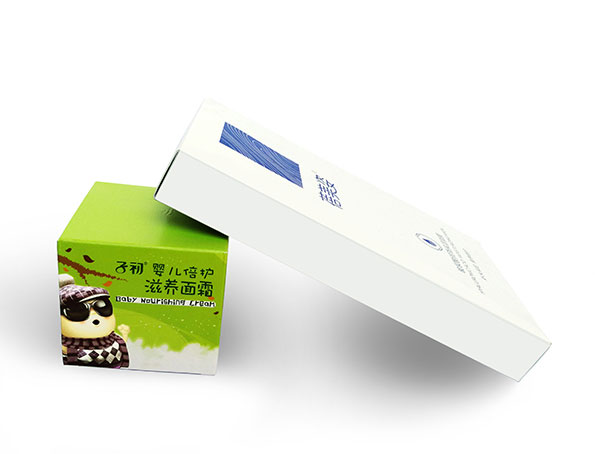## 印刷厂怎样计算画册书脊厚度书脊厚度如何计算？书脊在平面设计中是必定遇到的。如何计算一本画册或书刊的书脊位？相信很多设计师是不知如何计算。或是计算不准确。为了能计算准确的书脊位，避免后续反复修改尺寸等问题。设计师必需要了解几个要素：画册的P数、画册用纸，画册用纸克数、画册装订方式、纸张厚度。
例如：一本画册共60P，封面4P用250克双铜纸，内文56P用157克双铜纸，锁线胶装。
56÷2X0.13mm（内文157克双铜纸厚度）=3.64mm
4÷2X0.25mm（封面250克双铜纸厚度）=0.5mm
书脊厚度：3.64+0.5+1.5(锁线和胶水厚度)=5.64mm
若是无线胶装的不需加1.5mm的锁线位
计算出书脊位大小，设计师们就可以定封面的尺寸设计了。

#### 印刷常用纸厚度表(单位：mm)

 双铜纸 哑粉纸 双胶纸 80g=0.06 80g=0.07 50g=0.07 105g=0.08 105g=0.10 60g=0.08 128g=0.11 128g=0.12 70g=0.085 157g=0.13 157g=0.14 80g=0.095 200g=0.18 200g=0.21 100g=0.12 250g=0.25 250g=0.26 120g=0.14 300g=0.27 300g=0.32 160g=0.18 350g=0.37 350g=0.41 180g=0.22 400g=0.45 200g=0.25 230g=0.28 250g=0.32

### 【精彩文章】换一换